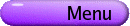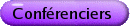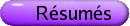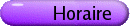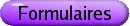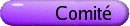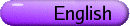Géométrie discrète et computationnelle
Org: Leroy J. Dickey (Waterloo) et Asia Ivic Weiss (York)
[PDF]

LEAH BERMAN, Ursinus College, Department of Mathematics & Computer Science, PO Box 1000, Collegeville, PA 19426, USA
Odd Astral Configurations
[PDF]

An astral configuration (pq, nk) is a collection of p points and n straight lines, usually in the Euclidean plane, where every point is incident with q straight lines, every line is incident with k points, and there are precisely ë[(q+1)/2] û symmetry classes (transitivity classes) of lines and ë[(k+1)/2] û symmetry classes of points. An odd astral configuration is an astral configuration where at least one of q and k is odd. This talk will discuss current known results about the existence of odd astral configurations where q and k are at least 4.

TED BISZTRICKY, University of Calgary
On edge-antipodal polytopes
[PDF]

A convex polytope is edge-antipodal if any two vertices,that determine an edge of the polytope, lie on distinct parallel supporting hyperplanes of the polytope. We survey recent results about such polytopes of dimension three or four.

JÜRGEN BOKOWSKI, Darmstadt University of Technology, Germany
Haskell and the Theory of Oriented Matroids
[PDF]

The use of functional programming within the theory of oriented matroids helps very much to deepen the understanding of various data structures within this fundamental part of Discrete Geometry. The author uses the functional programming language "Haskell" and main aspects of his forthcoming book, Computational Oriented Matroids (Cambridge University Press, 2005), to underline the above assertion. His intention is to invite the novice, perhaps even a novice in both disciplines, in Functional Programming and in the Theory of Oriented Matroids, to understand the benefit of putting these disciplines to work together.

With a 3-page list of basic Haskell functions, we cannot only write the Sieve of Erastostenes for finding prime numbers as an executable line ps = sieve[2...] where sieve(p:ns) = p:sieve[n | n < -ns, n¢mod¢p > 0], we can also reduce the burden of dealing with sign vectors that seem to have no geometric meaning for the novice.

ROBERT DAWSON, Saint Mary's University, Halifax, Nova Scotia
Non-Edge-to-Edge Triangulations of the Sphere
[PDF]

The tilings of the sphere with congruent spherical triangles meeting edge-to-edge were enumerated under certain assumptions by Sommerville in 1923, and completely by Davies in 1965. If we do not require the triangles to meet edge-to-edge, further tilings are possible. In this talk I will report (with plenty of pictures) on a probably-complete enumeration of spherical triangles that tile in such a fashion.

LEE DICKEY, University of Waterloo
Steiner Conics
[PDF]

Given a Pappus configuration, six distinct points 3 by 3, on two distinct lines, we find six different Pappus lines by permuting the points of each 3 set. These Pappus lines meet by threes in two points, which we call Steiner points. We study the conic that is the locus of such a Steiner point as a function of any one of the original six points.

BOB ERDAHL, Mathematic and Statistics, Queen's University
Voronoi's Conjecture on Parallelotopes
[PDF]

A parallelotope is a polytope with the following, very restrictive, combinatorial property: a selection of translates of the polytope fit together facet-to-facet to tile space. It is easy to show that the centers of the parallelotopes of such a tiling form a lattice. On the other hand, starting with a geometric lattice, the Voronoi polytope is determined by the Euclidean metric: the Voronoi polytope for a particular lattice point is the set of points as close to that lattice point as to any other. The Voronoi polytopes for all lattice points fit together facet-to-facet to tile space, so a Voronoi polytope is a special type of parallelotope. Voronoi conjectured in 1909 that these two tilings, definded is such different ways, are in fact equivalent; Voronoi conjectured each parallelotope tiling is affinely equivalent to a Voronoi tiling.

I will report on the recent work of Andrei Ordine who established that the Voronoi Conjecture holds for a new special case-for the case where the parallelotope is 3-irreducible, a condition that will be defined during the course of my lecture. I will also review the status of the Voronoi Conjecture, describing the various cases for which the Conjecture has been proven to hold, and discuss prospects for further developments.

Low Dimensional Neighbourly Polytopes
[PDF]

Among the d-polytopes with v vertices, the neighbourly polytopes have the greatest number of facets. This maximum property of neighbourly polytopes has prompted researchers to compile lists of them. In this talk, we will discuss the simplicial neighbourly 5-polytopes with nine vertices. We show that there are at least one hundred, twenty-six of them. We discuss the connection between the neighbourly 4-polytopes with eight vertices, the neighbourly 5-polytopes with nine vertices, and the neighbourly 6-polytopes with ten vertices.

CHRIS FISHER, University of Regina, Regina, SK S4S 0A2
Fourier Series and Ovals in Finite Geometry
[PDF]

A century ago, Hurwitz showed how Fourier series can be used to study geometric properties of curves in the Euclidean plane that bound convex sets. Ten years ago, Helmut Groemer wrote a book on the subject-Geometric Applications of Fourier Series and Spherical Harmonics. It turns out to be convenient to enlarge the scope to include all oriented smooth closed curves (in the plane) that have, for 0 £ f £ 2p, exactly one point where its directed tangent makes an angle of f with the positive x-axis. Such a curve has a parametrization z(f) : R ®C, where z(f) = z(f+2p) and the unit tangent vector is eif for all f Î R. These curves form a vector space over R that properly contains the boundaries of convex sets. Since many of the properties of such curves that one can investigate using Fourier series involve only algebra, much remains valid when R and C are replaced by some other field and its quadratic extension. In particular, I am interested in the geometry of the planes represented by the finite field GF(q2)-for x and y elements of the finite field GF(q) of order q, the point (x,y) corresponds to z=x+iy, where i is an element of GF(q2) that is not in GF(q). In fact, there is a point to this generalization: the use of finite Fourier series sheds light on a 50-year-old problem of Beniamino Segre, who sought a way to classify the ovals of finite projective planes. An oval is defined to be a set of q+1 points, no three on a line; it can be represented by a Fourier series that resembles that of its continuous kin.

ISABEL HUBARD, York University
Twisting self-dual chrial 4-polytopes
[PDF]

A chrial polytope is a non reflexible polytope with maximal symmetry by rotation. Self-dual chiral polytopes can be properly or improperly. Properly self-dual chrial 4-polytopes can be twisted to obtain regular maps. A similar operation can be done for improperly self-dual chiral 4-polytopes which will give us chiral quotents of the Petrie-Coxeter polyhedra.

LILY MOSHE, York University, 4700 Keele St., Toronto, ON M3J 1P3
Duality in Inductive Constructions of Circuits in 2-Rigidity via Tree Partitions
[PDF]

Given a graph G=(V,E), a realization in the plane G(p), as a framework, may be first-order rigid or first-order flexible. A basis is a minimal first-order rigid framework, while a circuit is a minimally redundant framework. For all generic realizations, the behaviour in the plane is determined by the combinatorics of the graph G. In this talk, we will investigate inductive constructions for extending a circuit to a larger circuit, for graphs on |V| vertices which are rigid for generic realizations in the plane.

Using Crapo's characterization of circuits in terms of two spanning trees, we explore some global properties of the circuits in terms of local changes in the graphs around a few vertices. For circuits on planar graphs, these inductions have a striking duality. The plane dual of a 2-circuit is a 2-circuit. This duality pairs techniques in a direct fashion and offers additional insights and corollaries.

This talk is based on joint work with Walter Whiteley and Laura Chávez Lomelí.

TOMAZ PISANSKI, IMFM, University of Ljubljana and University of Primorska
Dimension of unsplittable incidence structures
[PDF]

A combinatorial incidence structure C = (P,L,I) consists of 'points' P, 'lines' L, and an incidence relation I between them. The corresponding bipartite incidence graph is sometimes called the Levi graph of the structure.

A collection of points and lines in the Euclidean space defines a geometric incidence structure. Each geometric incidence structure determines a unique combinatorial incidence structure. The converse is not true. An incidence-preserving mapping of 'points' and 'lines' of a combinatorial incidence structure C to points and lines of an Euclidean space is called a representation of C.

dim(C) is the maximum dimension of the affine span of any geometric incidence structure representing combinatorial incidence structure C.

G-graph is the graph square of the Levi graph of an incidence structure C. An incidence structure C is unsplittable if by removing any set of vertices independent in G-graph from the Levi graph of C, the Levi graph remains connected. This talk will indicate some problems concerning the dimension of unsplittable incidence structures in particular for some combinatorial or geometric configurations.

This is joint work in progress with Branko Grünbaum.

DORETTE PRONK, Department of Mathematics and Statistics, Dalhousie University, Halifax, NS B3H 3J5
Touching Wood-the Shape of Fractal Trees
[PDF]

In this talk we study non-overlapping symmetric binary fractal trees as representations of the free monoid M(L,R) on two generators L and R. Such a tree is determined by an angle q and a scaling ratio r, such that the interiors of its branches do not overlap.

Motivated by techniques from shape theory and computational topology we consider for each fractal tree the homology of its inverse system of closed e-neighbourhoods. We show that holes in these e-neighbourhoods are determined by certain pairs of "contact vertices" on the tree and use this to identify different types of holes.

This leads us to consider a coloured version of the "topological barcode" (as introduced by Carlsson et al. in ) of a fractal tree. The topological barcode encodes for each hole its persistence interval, that is, the interval of the epsilon values for which this hole exists. Moreover, there is a natural action of the monoid M(L,R) on the coloured barcode. This will lead to several new classifications of symmetric binary fractal trees. Tara Taylor will discuss this in more detail in her presentation in this session, including several interesting "golden" examples.

This is joint work with Tara Taylor (St. Francis Xavier University).

## References


G. Carlsson, A. Zomorodian, A. Collins and L. Guibas, Persistence barcodes for shapes. In: Symposium on Geometry Processing, Nice, France, July 8-10, 2004 (can also be found on the website math.stanford.edu/comptop/preprints/).

FRANCO SALIOLA, Cornell University, Ithaca, NY, USA
Geometry and Algebra associated to Hyperplane Arrangements
[PDF]

The geometry and combinatorics of hyperplane arrangements define an associative product on the faces of the arrangement and is used in determining various properties of the resulting semigroup algebra. In turn, the properties of the algebra afford geometric and combinatorial results about the arrangement. An example of this interplay is the result that the face semigroup algebra depends only on the intersection lattice of the hyperplane arrangement. It is obtained by constructing the quiver of the face semigroup algebra using the geometry of the hyperplane arrangement.

EGON SCHULTE, Northeastern University, Department of Mathematics, Boston, MA 02115, USA
Reflection groups and polytopes over finite fields
[PDF]

Any finite or infinite Coxeter group G with string diagram is the automorphism group of an abstract regular polytope. When G is crystallographic, its standard real representation is easily reduced modulo an odd prime p, thus giving a finite representation in some finite orthogonal space V over the field with p elements. The finite group need not be polytopal; and whether or not it is depends in an intricate way on the geometry of V. The talk presents recent work with Barry Monson, in which we describe this construction in considerable generality and study in depth the interplay between the geometric properties of the polytope (if it exists) and the algebraic structure of the overlying finite orthogonal group. The rank 4 case has been worked out in considerable detail.

TARA TAYLOR, St. Francis Xavier University, Antigonish, Nova Scotia
Finding Gold in the Forest: Fractal Trees and The Golden Ratio
[PDF]

This talk will expand on the talk presented by Dorette Pronk of Dalhousie University (in the same session). We continue with a discussion of the classifications of symmetric binary fractal trees via an analysis of the closed e-neighbourhoods using methods of computational topology. We illustrate the theory we have developed to study fractal trees by discussing an overview of the topology of four self-contacting trees that are related to the golden ratio. These four trees each possess remarkable symmetry, and their topological barcodes demonstrate the rich structure that a topological barcode can add to a fractal tree.

ASIA IVIC WEISS, York University
Gray graph as medial graph of a 4-polytope
[PDF]

Each combinatorial polytope of rank 4 which can be assigned Schläfli symbol {k,q,k} yields a bipartite k-valent graph, called medial graph of the polytope, whose vertices are the faces of the polytope of ranks 1 and 2. Two vertices of such graph are adjacent whenever the corresponding faces are incident. I shall present recent work with Barry Monson, in which we prove that the medial graph for the polytope with regular toroidal facets {3,6}(3,0) and vertex-figures {6,3}(1,1) is an edge-transitive graph with 54 vertices, known as the Gray graph. In fact, our construction yields an infinite family of edge-transitive graphs which are not vertex-transitive. Gray graph is the smallest known graph with this property.

WALTER WHITELEY, York University, Toronto, Ontario
Some applications of rigidity to control of formations
[PDF]

Recent work on control of formations of robots has used a number of results from rigidity theory, while adding some new questions about directed graphs (which of the end vertex agents is responsible for maintaining the length of the edge?). In addition to graphs which can be rigidly maintained, there are problems of `control'-will noise disrupt the convergence of the formation to the desired path?

We will present some key results drawn from rigidity theory and as well as currrent unsolved problems for formations of agents in the plane, with distance constraints.

This is part of joint work with groups of Steven Morse (Electrical Engineering, Yale), Richard Yang (Computer Science, Yale) and Peter Belhumeur and Tolga Eren (Computer Science, Columbia).

GORDON WILLIAMS, Ursinus College, PO Box 1000, Collegeville, PA 19426, USA
Geometric Realizations of Non-Convex Simplicial Spheres
[PDF]

The study of combinatorial types of convex polytopes has been an extremely fruitful one for twentieth-century mathematics. In 1967, Grünbaum and Shreedharan discovered some errors in Brückner's 1909 enumeration of the simple convex polytopes with 8 facets in 4-dimensional Euclidean space. In particular, they discovered that one of the combinatorial types listed by Brückner did not admit a realization as the boundary of a convex polytope. Since then, much work has been done on determining which simplicial spheres admit realizations as the boundaries of convex polytopes; we will call such spheres convexly realizable. This talk will review some of that work and explore some of the interesting questions that arise when one begins to investigate the geometric structure of those simplicial spheres that are not convexly realizable.